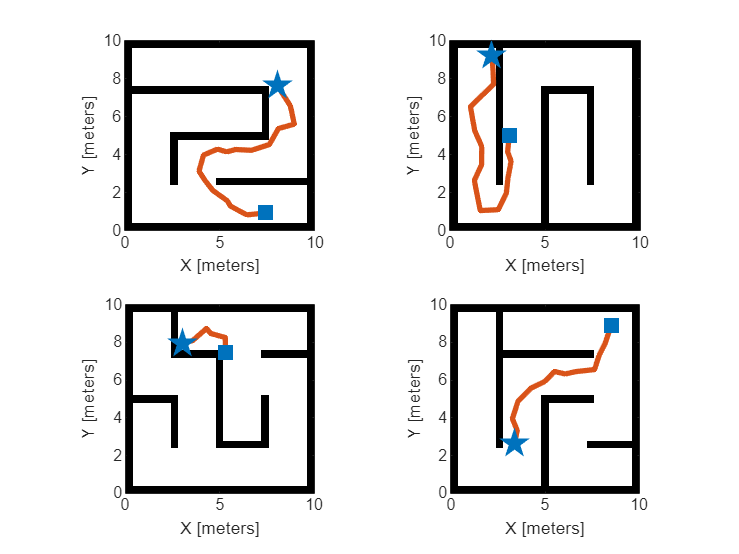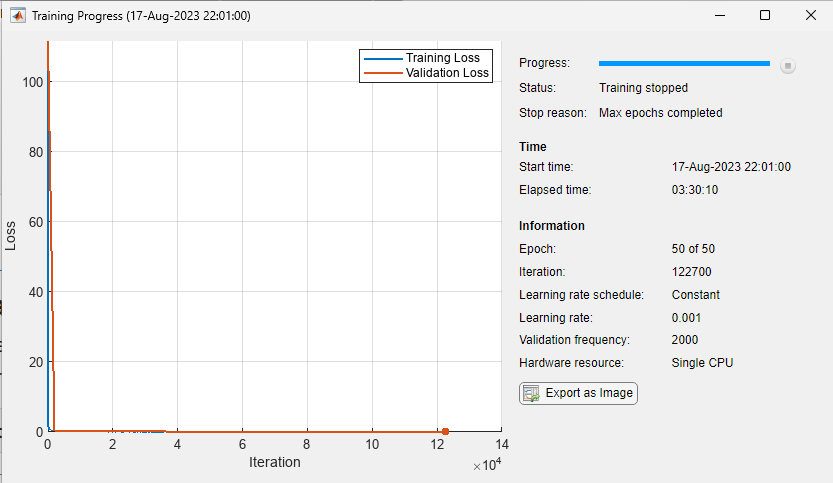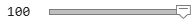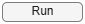# Train Deep Learning-Based Sampler for Motion Planning

This example shows how to create a deep learning-based sampler using Motion Planning Networks to speed up path planning using sampling-based planners like RRT (rapidly-exploring random tree) and RRT*. For information about Motion Planning Networks (MPNet) for state space sampling, see Get Started with Motion Planning Networks (Navigation Toolbox).

### Load and Visualize Training Data set

Load the data set from a .`mat` file. The data set contains 400,000 different paths for 200 maze map environments. The data set has been generated for a pre-defined parameters of `mazeMap` function. The first column of the data set contains the maps and the second column contains the optimal paths for randomly sampled start, goal states from the corresponding maps. The size of data set is 75MB.

```% Download and extract the maze map dataset if ~exist("mazeMapDataset.mat","file") datasetURL = "https://ssd.mathworks.com/supportfiles/nav/data/mazeMapDataset.zip"; websave("mazeMapDataset.zip", datasetURL); unzip("mazeMapDataset.zip") end % Load the maze map dataset load("mazeMapDataset.mat","dataset","stateSpace") head(dataset)```
``` Map Path ______________________ _____________ 1×1 binaryOccupancyMap {14×3 single} 1×1 binaryOccupancyMap { 8×3 single} 1×1 binaryOccupancyMap {24×3 single} 1×1 binaryOccupancyMap {23×3 single} 1×1 binaryOccupancyMap {17×3 single} 1×1 binaryOccupancyMap {15×3 single} 1×1 binaryOccupancyMap { 7×3 single} 1×1 binaryOccupancyMap {10×3 single} ```

The data set was generated using the `examplerHelperGenerateDataForMazeMaps` helper function. The `examplerHelperGenerateDataForMazeMaps` helper function uses the `mapMaze` function to generate random maze maps of size 10-by-10 and resolution 2.5 m. The width and wall thickness of the maps was set to 5m and 1 m, respectively.

```passageWidth = 5; wallThickness = 1; map = mapMaze(passageWidth,wallThickness,MapSize=[10 10],MapResolution=2.5) ```

Then, the start states and goal states are randomly generated for each map. The optimal path between the start and goal states are computed using `plannerRRTStar` path planner. The `ContinueAfterGoalReached` and `MaxIterations` parameters are set to `true` and `5000`, respectively, to generate the optimal paths.

```planner = plannerRRTStar(stateSpace,stateValidator); % Uses default uniform state sampling planner.ContinueAfterGoalReached = true; % Optimize after goal is reached planner.MaxIterations = 5000; % Maximum iterations to run the planner ```

Visualize a few random samples from the training data set. Each sample contains a map and the optimal path generated for a given start and goal state.

```figure for i=1:4 subplot(2,2,i) ind = randi(height(dataset)); % Select a random sample map = dataset(ind,:).Map; % Get map from Map column of the table pathStates = dataset(ind,:).Path{1}; % Get path from Path column of the table start = pathStates(1,:); goal = pathStates(end,:); % Plot the data show(map); hold on plot(pathStates(:,1),pathStates(:,2),plannerLineSpec.path{:}) plot(start(1),start(2),plannerLineSpec.start{:}) plot(goal(1),goal(2),plannerLineSpec.goal{:}) title("") end legend```You can modify the helper function to generate new maps and train the MPNet from scratch. The dataset generation may take a few days depending upon CPU configuration and the number of maps you want to generate for training. To accelerate dataset generation, you can use Parallel Computing Toolbox™.

### Create Motion Planning Networks

Create Motion Planning Networks (MPNet) object for SE(2) state space using `mpnetSE2`.

`mpnet = mpnetSE2;`

Set the `StateBounds`, `LossWeights`, and `EncodingSize` properties of the `mpnetSE2` object. Set the `StateBounds` using the `StateBounds` property of the `stateSpace` object.

`mpnet.StateBounds = stateSpace.StateBounds;`

The loss function is the weighted mean squared loss computed between a predicted next state and the actual state (ground truth) taken from the data set. You must specify the weights for each state space variable $\mathit{x}$, $\mathit{y}$, and $\theta$ of SE(2) state space. For a SE(2) state space, we do not consider the robot kinematics such as the turning radius. Hence, you can assign zero weight value for the $\theta$ variable.

Specify the weights for each state space variables using the `LossWeights` property of the `mpnetSE2` object.

`mpnet.LossWeights = [100 100 0];`

Specify the value for `EncodingSize` property of the `mpnetSE2` object as [9 9]. Before training the network, the `mpnetSE2` object encodes the input map environments to a compact representation of size 9-by-9.

`mpnet.EncodingSize = [9 9];`

### Prepare Data for Training

Split the dataset into train and test sets in the ratio 0.8:0.2. The training set is used to train the `Network` weights by minimizing the training loss, validation set is used to check the validation loss during the training.

```split = 0.8; trainData = dataset(1:split*end,:); validationData = dataset(split*end+1:end,:);```

Prepare the data for training by converting the raw data containing the maps and paths into a format required to train the MPNet.

```dsTrain = mpnetPrepareData(trainData,mpnet); dsValidation = mpnetPrepareData(validationData,mpnet);```

Visualize prepared dataset. The first column of sample contains the encoded map data, encoded current state and goal states. The second column contains the encoded next state. The encoded state is computed as $\left[x,y,\mathrm{cos}\left(\theta \right),\mathrm{sin}\left(\theta \right)\right]$ and normalized to the range of `[0, 1]`.

```preparedDataSample = read(dsValidation); preparedDataSample(1,:)```
```ans=1×2 cell array {[0.2607 0.4112 0.6846 0.9647 0.9138 0.5757 0.4883 1.3733e-04 0.0549 0.1646 0 0.1646 0.1646 0.1646 0.1646 0.1646 0.0549 0.1646 0.8244 0.0870 0.9383 0.8244 0.8244 0.8244 0.8244 0.1646 0.1646 0.8244 0.0870 0.9020 0.0094 0.0870 0.0870 0.0870 3.9316e-16 0.1646 0.8244 0.0870 0.9020 0.0094 0.9020 0.9043 0.9383 0.1646 0.1646 0.8244 0.0870 0.9020 0.0094 0.9020 0.0870 0.8244 0.1646 0.1646 1 0.9043 0.9020 0.0094 0.9020 0.0870 0.8244 0.1646 0.1646 0.8313 0.0870 0.0870 0.0094 0.9020 0.0870 0.8244 0.1646 0.1646 0.9333 0.8244 0.8244 0.8244 0.9383 0.0870 0.8244 0.1646 0.0549 0.1646 0.1646 0.1646 0.1646 0.1646 2.6928e-16 0.1646 0.0549]} {[0.2720 0.4130 0.6786 0.9670]} ```

### Train Deep Learning Network

Use the `trainnet` function to train the MPNet. Training this network might take a long time depending on the hardware you use. Set the `doTraining` value to `true` to train the network.

`doTraining = false;`

Specify `trainingOptions` for training the deep learning network:

• Set the `MiniBatchSize` for training to 2048.

• Shuffle the `dsTrain` at every epoch.

• Set the `MaxEpochs` to 50.

• Set the `ValidationData` to `dsValidation` and `ValidationFrequency` to 2000.

You can consider the training to be successful once the training loss and validation loss converge close to zero.

```if doTraining options = trainingOptions("adam",... MiniBatchSize=2048,... MaxEpochs=50,... Shuffle="every-epoch",... ValidationData=dsValidation,... ValidationFrequency=2000,... Plots="training-progress"); % Train network [net, info] = trainnet(dsTrain, mpnet.Network, @mpnet.loss, options); % Update Network property of mpnet object with net mpnet.Network = net; end```Alternatively, you can directly use a pretrained MPNet to compute predictions.

Load a `.mat` file containing the pretrained network. The network has been trained on various, randomly generated maze maps stored in the `mazeMapDataset.mat` file.

```if ~doTraining load("mazeMapTrainedMPNET.mat","trainedNetwork") mpnet.Network = trainedNetwork; end```

### Create MPNet State Sampler

Create a MPNet state sampler using the state space and the MPNet object as inputs. You can update the `Environment`, `StartState`, and `GoalState` properties to generate samples for new scenarios.

`stateSamplerDL = stateSamplerMPNET(stateSpace,mpnet);`

### Generate Learned Samples on Validation Data

Press the `Run` button below to generate learned samples for different samples in the test data set.

```% Get random sample from testData ind = randi(height(validationData)); map = validationData(ind,:).Map; start = double(validationData(ind,:).Path{1,:}(1,:)); goal = double(validationData(ind,:).Path{1,:}(end,:));```

Set the `Environment`, `StartState,` `GoalState`, `MaxLearnedSamples` properties for the `stateSampler`.

```stateSamplerDL.Environment = map; stateSamplerDL.StartState = start; stateSamplerDL.GoalState = goal;```

You can vary total samples generated by the sampler by adjusting `numSamples`. You can also vary `MaxLearnedSamples(<=numSamples)` to visualize the mix of learned samples and uniform samples.

```numSamples =100; stateSamplerDL.MaxLearnedSamples =100;```

Press `Run` button below to generate samples for a new scenario.

```samples = sample(stateSamplerDL, numSamples);```

Visualize generated samples

```figure show(map); hold on; plot(samples(:,1),samples(:,2),plannerLineSpec.state{:}) plot(start(1),start(2),plannerLineSpec.start{:}); plot(goal(1),goal(2),plannerLineSpec.goal{:}); legend(Location="eastoutside")```### Conclusion

This example shows how to train a MPNet to generate learned samples for sampling-based planners such as RRT and RRT*. It also shows the data generation process, deep learning network setup, training, and prediction. You can modify this example to use with custom maps and custom datasets. Further, you can extend this for applications like manipulator path planning, 3-D UAV path planning, and more.

To augment sampling-based planners with the deep learning-based sampler to find optimal paths efficiently, See Accelerate Motion Planning with Deep-Learning-Based Sampler (Navigation Toolbox) example.